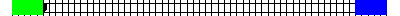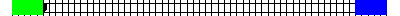# Two Trains and a Bee

Two trains are on the same track a distance 100 km apart heading towards one another, each at a speed of 50 km/h. A Bee starting out at the front of one train, flies towards the other at a speed of 75 km/h. Upon reaching the other train, the Bee turns around and continues towards the first train. How many kilometers does the Bee travel before getting squashed in the collision of the two trains?

Solution:Let the first train moves at u km/h
second train moves at v km/h
distance between trains be d km
speed of Bee is f km/h
time taken by trains to collide = d/(u+v)
distance travelled by bee = f*d/(u+v) = 75* 100/(50+50) = 75